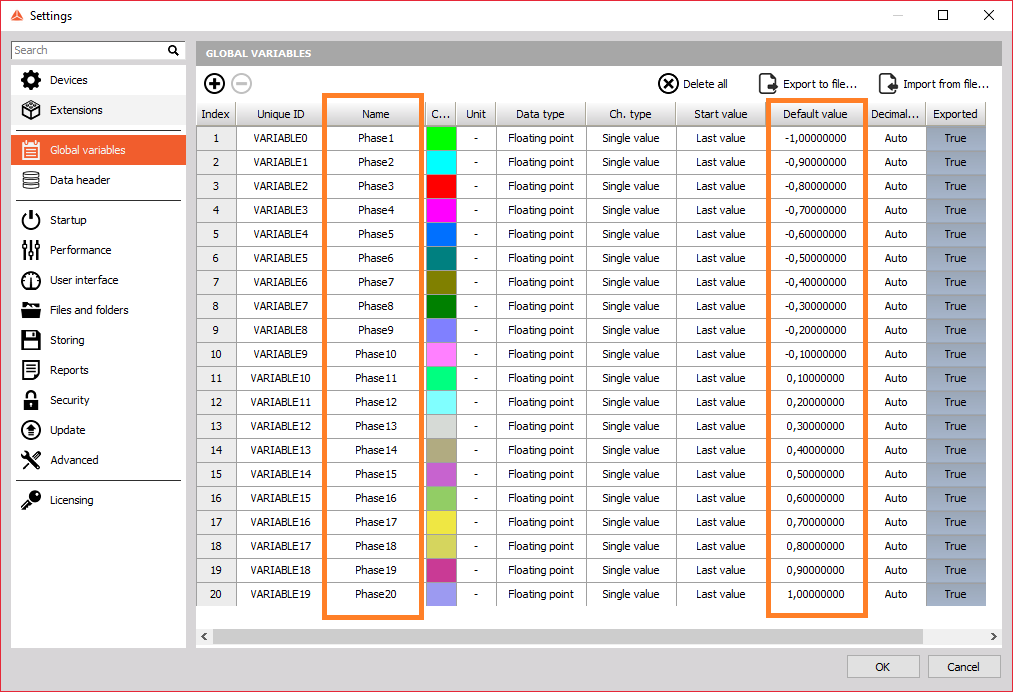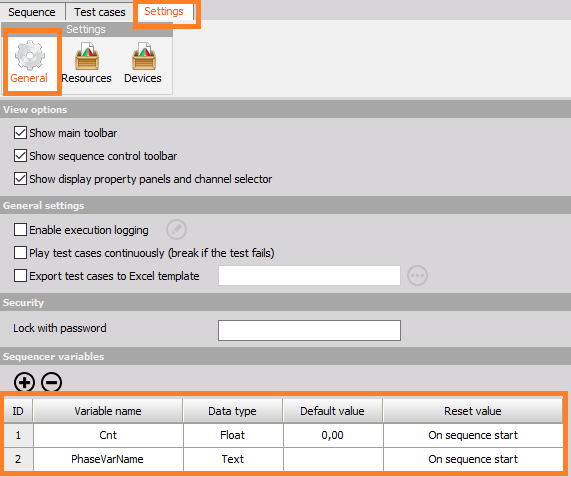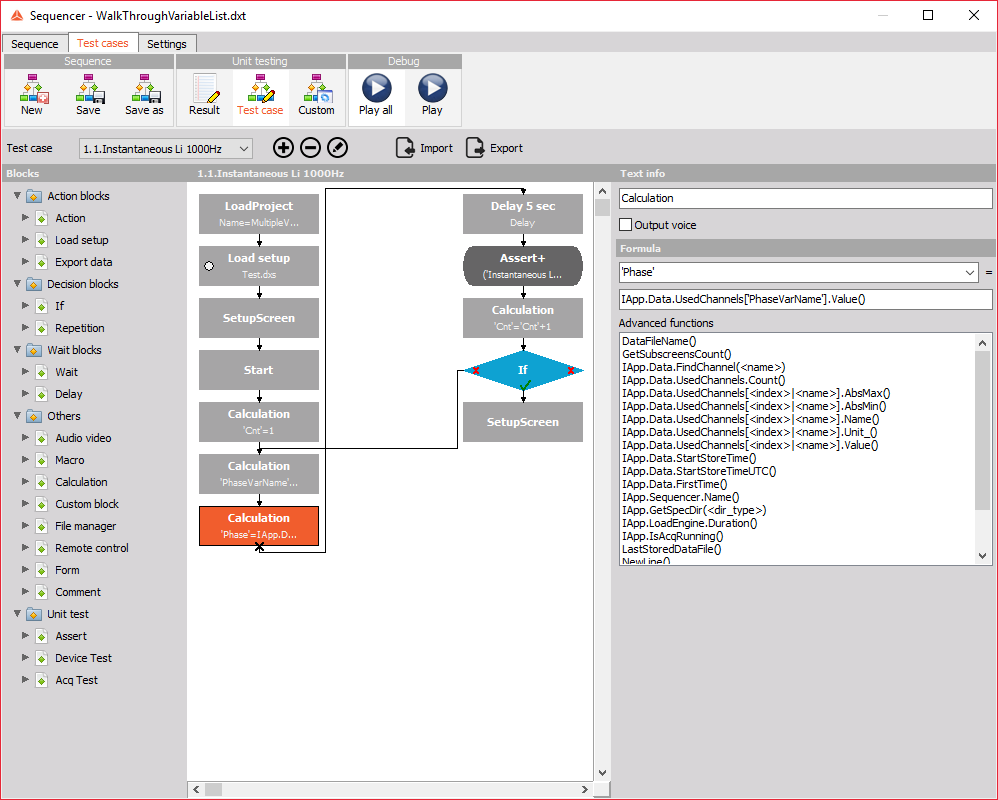This solution describes how to make a sequence, which goes through a list of variables. This is useful if you have an application, where for each measurement you need to change the value of a user input.

1. You need to make a project which has a list of global variables. All the variables' names should be the same, but with an increasing number in the end. In the sequence you need to have an action block which loads this project.2. In the sequencer general settings you need to define the variables. The first variable should be for the counter, which will go through the list and it needs to be a float type. The second variable needs to be a text type and it will be used to combine the text part of the variable name and the counter to select a specific global variable.3. In the sequencer, we first add an action block to load the project and the second one to load a setup with a user input which will use the global variables.
4. The next block is a calculation block in which we need to set the counter so it will use our first variable, in our case 1.5. In the next calculation block we combine the text with the current counter number, to get the correct variable name. We write this name to our sequencer variable, which we defined in the sequence settings.6. In the last calculation block we use the value of our global variable in our user input. In this example, we have a user input in our setup called Phase. With the "IApp.Data.UsedChannels['PhaseVarName'].Value()" function, we set the user input Phase to have the value of the global variable Phase1 which is -1. Remember that the value of the sequence variable 'PhaseVarName' is now Phase1 and the function "IApp.Data.UsedChannels[<index>|<name>].Value()" gives us the value of the variable Phase1.7. This phase will be used for 5 seconds, then we increase the counter by 1 using the calculation block with the formula 'Cnt'='Cnt'+1. We use an if decision block, to check if we are at the end of the list. If we aren't we go back to the calculation block which forms the variable name and if we are we finish with the sequence.